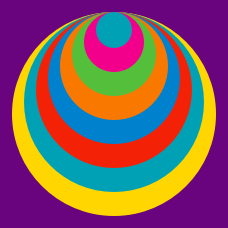Geometry

Composite Figures: Level 4 ChallengesIn the above diagram, each of the grid squares is a unit long. Also, all the arcs are quadrants. If the area of the shape enclosed by arcs $DB$, $BJ$, $JK$, and $KD$ can be represented as $\dfrac{a \pi + b}{c},$ where $a,b,c$ are integers with $c$ positive, find the maximum value of $a+b+c$.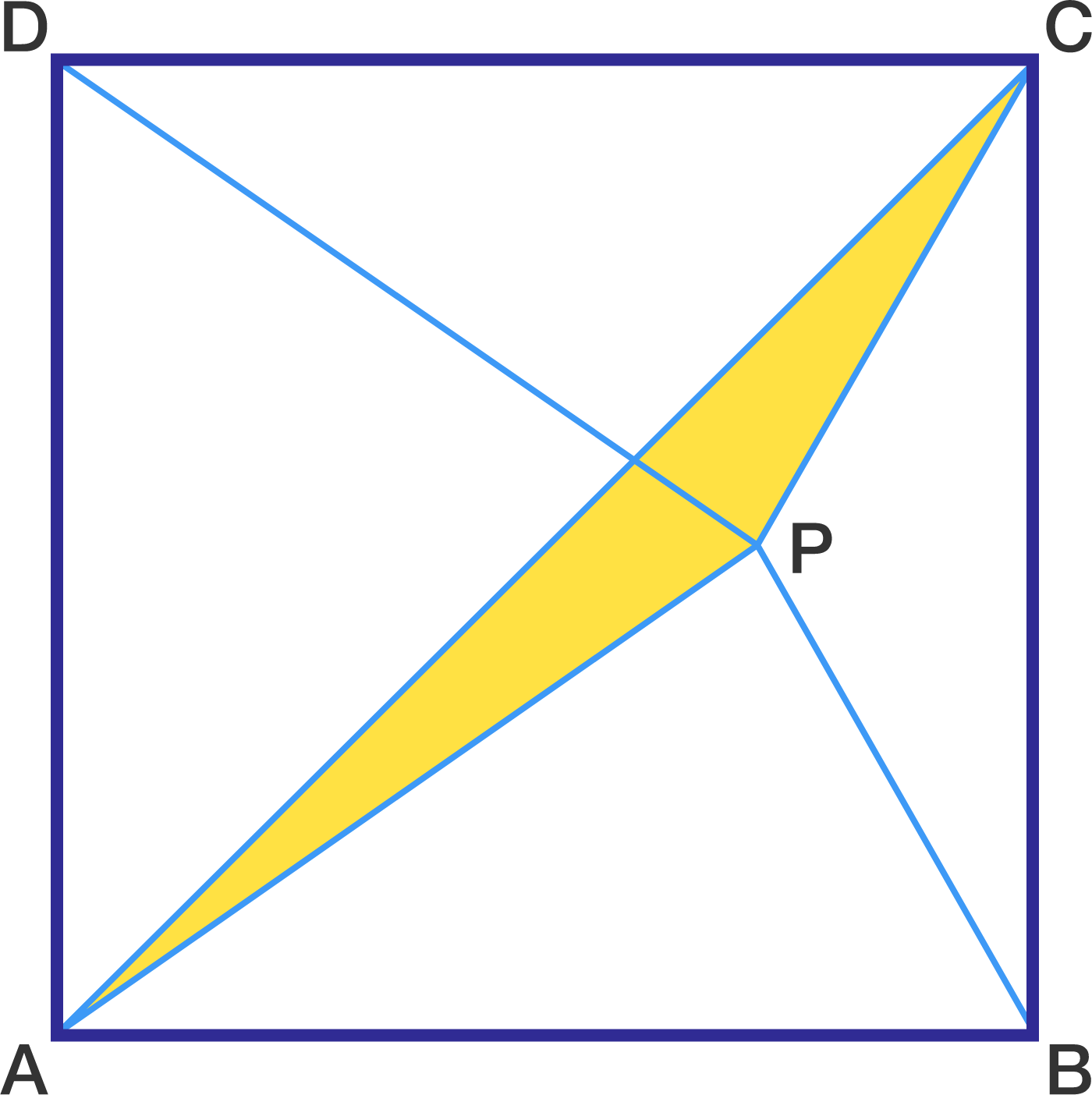Let $ABCD$ be a square and $P$ be a point inside the square such that $PB = 23$ and $PD = 29$ . Find the area of $\triangle APC$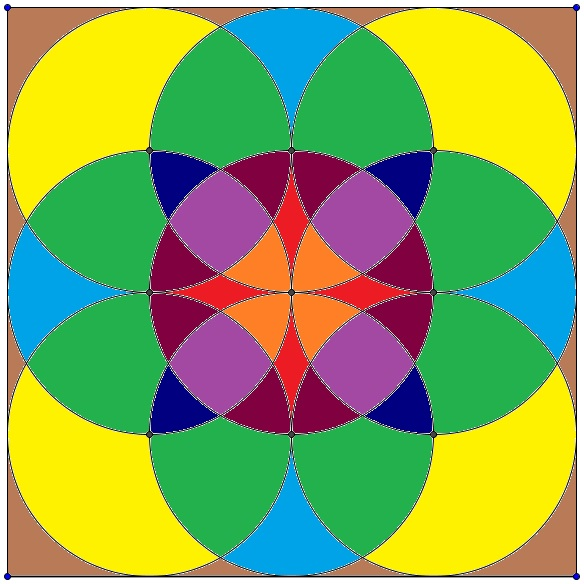Find the area of $\color{#BA33D6}{Violet}$ region (four square looking region of central circle) if radius of each circle is 4 units.

Image credit goes to Aniket Verma.

As shown, a rectangle (not a square) is partitioned into four triangles: 1 equilateral and 3 right triangles. The areas of two of the right triangles are 30 and 50. Find the area $x$ of the remaining right triangle.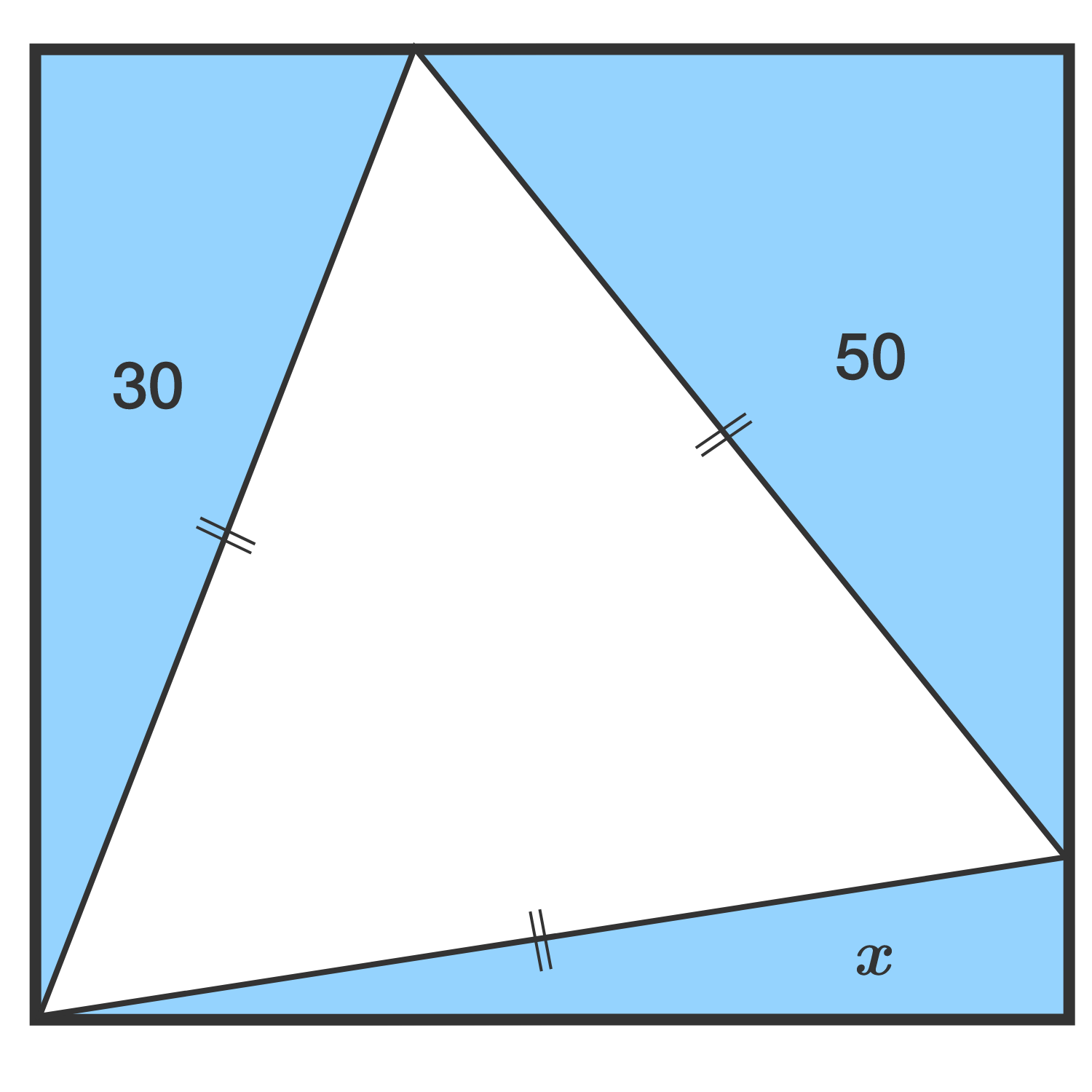Bonus: Find the general relationship among the areas of the 3 right triangles.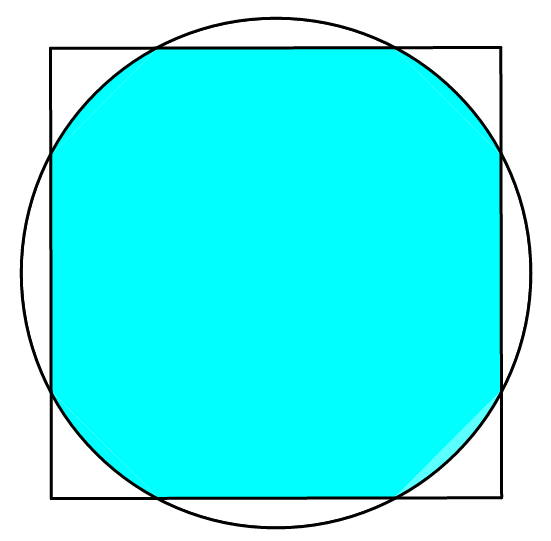Suppose we combine a unit circle and a square of the same area, as shown above, such that their centroids lie on the same point. What is the area of the non-overlapping white region?

If your answer is of the following form $A \arctan\left(\dfrac{\sqrt{\pi\left(B - \pi\right)}}{\pi - C}\right) - D \sqrt{\pi\left(E - \pi\right)},$ where $A, B, C, D,$ and $E$ are positive integers, evaluate $A + B + C + D + E$.

×